# Characteristics of an Ideal Filter (LPF, HPF, BPF and BRF)

## What is a Filter?

A filter is a frequency selective network, i.e., it allows the transmission of signals of certain frequencies with no attenuation or with very little attenuation and it rejects all other frequency components.

## What is an Ideal Filter?

An ideal filter is a frequency selective network that has very sharp cut-off characteristics, i.e., it transmits the signals of certain specified band of frequencies exactly and totally rejects the signals of frequencies outside this band. Therefore, the phase spectrum of an ideal filter is linear.

## Ideal Filter Characteristics

Based on the frequency response characteristics, the ideal filters can be of following types −

• Ideal Low-Pass Filter (LPF)

• Ideal High-Pass Filter (HPF)

• Ideal Band-Pass Filter (BPF)

• Ideal Band-Reject Filter (BRF)

• Ideal All Pass Filter

## Ideal Low Pass Filter (LPF)

An ideal low pass filter is the one which transmits all the signal of frequencies less than a certain frequency $\mathit{\omega_{c}}$ radians per second without any distortion and blocks all the signals of frequencies above $\mathit{\omega_{c}}$ radians per second. Where, the frequency $\mathit{\omega_{c}}$ radians per second is called the cut-off frequency. The phase function of an ideal low-pass filter is given by $[\mathit{\theta \left(\omega\right)=-\omega t_{d}}]$.

The transfer function of an ideal low-pass filter is given by,

$$\mathrm{\mathit{\left | H\left(\omega\right) \right |=\left\{\begin{matrix} \mathrm{1}\:\:\mathrm{for}\:\left | \omega\ \right|<\omega_{c} \ \mathrm{2} \:\:\mathrm{for}\:\left | \omega\ \right|>\omega_{c} \end{matrix}\right.}}$$

Therefore, the frequency response characteristics of an ideal low-pass filter is a gate or rectangular function and it is shown in Figure-1.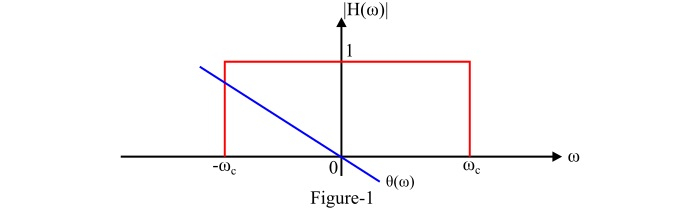## Ideal High Pass Filter (HPF)

An ideal high pass filter transmits all the signals of frequencies above a certain frequency $\mathit{\omega_{c}}$ radians per second without any distortion and blocks completely all the signals of frequencies below the frequency $\mathit{\omega_{c}}$ radians per second. Here, the frequency $\mathit{\omega_{c}}$ radians per second is called the cut-off frequency. The phase function of an ideal high pass filter is given by,

$$\mathrm{\theta (\omega )=-\omega t_{d}}$$

The transfer function of an ideal high pass filter is given by,

$$\mathrm{\mathit{\left | H\left(\omega\right) \right |=\left\{\begin{matrix} \mathrm{0}\:\:\mathrm{for}\:\left | \omega\ \right|<\omega_{c} \ \mathrm{1} \:\:\mathrm{for}\:\left | \omega\ \right|>\omega_{c} \end{matrix}\right.}}$$

Figure-2 shows the frequency response characteristics of an ideal high pass filter.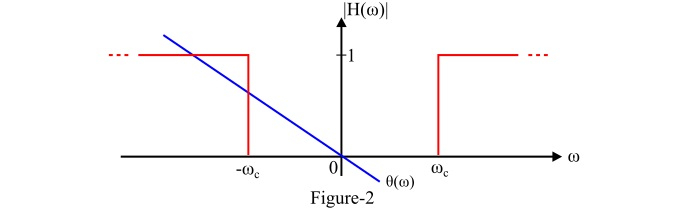## Ideal Band Pass Filter (BPF)

An ideal band pass filter transmits all the signals of frequencies within a certain frequency band $\mathit{\left(\omega _{\mathrm{2}}-\omega_{\mathrm{1}}\right)}$ radians per second without any distortion and completely blocks all the signals of frequencies outside this frequency band.

The frequency band $\mathit{\left(\omega _{\mathrm{2}}-\omega_{\mathrm{1}}\right)}$ is called the bandwidth of the band-pass filter.

The phase function of an ideal band-pass filter for the distortion less transmission is given by,

$$\mathrm{\mathit{\theta\left(\omega\right)=-\omega t_{d}}}$$

And the transfer function of an ideal band-pass filter is given by,

$$\mathrm{\mathit{\left | H\left(\omega\right) \right |=\left\{\begin{matrix} \mathrm{1}\:\:\mathrm{for}\:\left | \omega_{\mathrm{1}}\ \right|<\omega< \left | \omega_{2}\ \right| \\mathrm{0} \:\:\mathrm{for}\:\omega<\left | \omega_{\mathrm{1}}\ \right|\&\:\omega>\left | \omega_{\mathrm{2}}\ \right| \end{matrix}\right.}}$$

The figure-3 shows the frequency response characteristics of an ideal band-pass filter (BPF).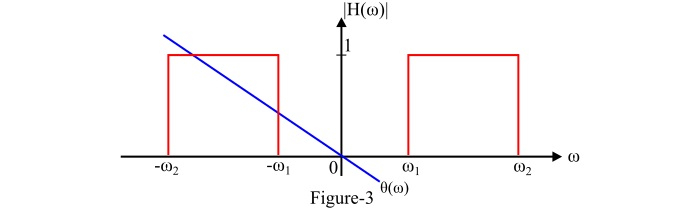## Ideal Band Rejection Filter (BRF)

An ideal band rejection filter rejects completely all the signals of frequencies within a frequency band $\mathit{\left(\omega _{\mathrm{2}}-\omega_{\mathrm{1}}\right)}$ radians per second and transmits all the signals of frequencies outside the frequency band without any distortion.

In this case, the frequency band $\mathit{\left(\omega _{\mathrm{2}}-\omega_{\mathrm{1}}\right)}$ is called the rejection band. The Band rejection filter is also called the band stop filter (BSF) or band-elimination filter (BEF). The phase function of an ideal band rejection filter is $\mathit{\theta\left(\omega\right)=-\omega t_{d}}$.

And the transfer function of an ideal band rejection filter is given by,

$$\mathrm{\mathit{\left | H\left(\omega\right) \right |=\left\{\begin{matrix} \mathrm{0}\:\:\mathrm{for}\:\left | \omega_{\mathrm{1}}\ \right|<\omega< \left | \omega_{\mathrm{2}}\ \right| \\mathrm{1} \:\:\mathrm{for}\:\omega<\left | \omega_{\mathrm{1}}\ \right|\&\:\omega>\left | \omega_{\mathrm{2}}\ \right| \end{matrix}\right.}}$$

The figure-4 shows the frequency response characteristics of an ideal band rejection filter.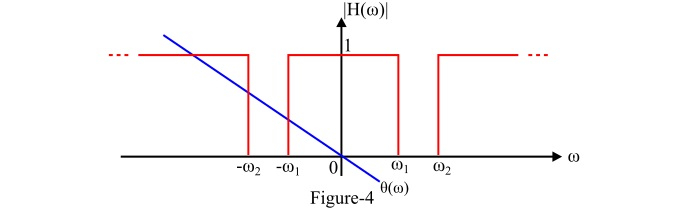## Ideal All Pass Filter

An all pass filter is a frequency selective network which transmits signals of all the frequencies without any distortion. That is, the bandwidth of an all pass filter is infinite as shown in figure-5. The transfer function of an ideal all pass filter is given by,

$$\mathrm{\mathit{\left| H\left(\omega\right)\right |=\left\{\mathrm{1}\:\:\mathrm{for} \:\mathrm{all}\:\omega\right.}}$$

And the phase function of an ideal all pass filter for the distortion less transmission is $\mathit{\theta\left(\omega\right)=-\omega t_{d}}$.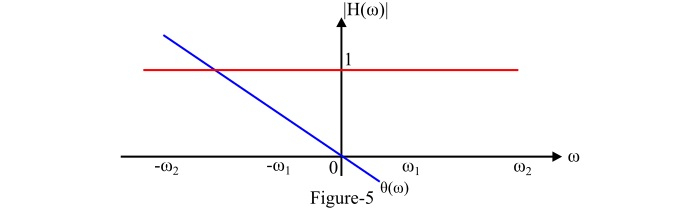Note - All ideal filters are non-causal systems. Therefore, none of them can be physically realizable.